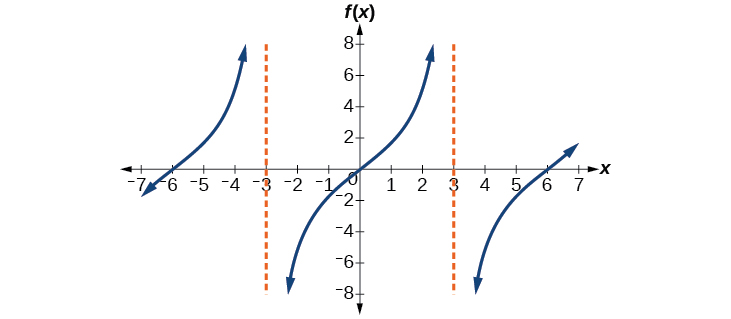# 8.2 Graphs of the other trigonometric functions  (Page 2/9)

 Page 2 / 9

## Graphing variations of y = tan x

As with the sine and cosine functions, the tangent    function can be described by a general equation.

$y=A\mathrm{tan}\left(Bx\right)$

We can identify horizontal and vertical stretches and compressions using values of $\text{\hspace{0.17em}}A\text{\hspace{0.17em}}$ and $\text{\hspace{0.17em}}B.\text{\hspace{0.17em}}$ The horizontal stretch can typically be determined from the period of the graph. With tangent graphs, it is often necessary to determine a vertical stretch using a point on the graph.

Because there are no maximum or minimum values of a tangent function, the term amplitude cannot be interpreted as it is for the sine and cosine functions. Instead, we will use the phrase stretching/compressing factor when referring to the constant $\text{\hspace{0.17em}}A.$

## Features of the graph of y = A Tan( Bx )

• The stretching factor is $\text{\hspace{0.17em}}|A|.$
• The period is $\text{\hspace{0.17em}}P=\frac{\pi }{|B|}.$
• The domain is all real numbers $\text{\hspace{0.17em}}x,$ where $\text{\hspace{0.17em}}x\ne \frac{\pi }{2|B|}+\frac{\pi }{|B|}k\text{\hspace{0.17em}}$ such that $\text{\hspace{0.17em}}k\text{\hspace{0.17em}}$ is an integer.
• The range is $\text{\hspace{0.17em}}\left(\mathrm{-\infty },\infty \right).$
• The asymptotes occur at $\text{\hspace{0.17em}}x=\frac{\pi }{2|B|}+\frac{\pi }{|B|}k,\text{\hspace{0.17em}}$ where $\text{\hspace{0.17em}}k\text{\hspace{0.17em}}$ is an integer.
• $y=A\mathrm{tan}\left(Bx\right)\text{\hspace{0.17em}}$ is an odd function.

## Graphing one period of a stretched or compressed tangent function

We can use what we know about the properties of the tangent function to quickly sketch a graph of any stretched and/or compressed tangent function of the form $\text{\hspace{0.17em}}f\left(x\right)=A\mathrm{tan}\left(Bx\right).\text{\hspace{0.17em}}$ We focus on a single period    of the function including the origin, because the periodic property enables us to extend the graph to the rest of the function’s domain if we wish. Our limited domain is then the interval $\text{\hspace{0.17em}}\left(-\frac{P}{2},\frac{P}{2}\right)\text{\hspace{0.17em}}$ and the graph has vertical asymptotes at $\text{\hspace{0.17em}}±\frac{P}{2}\text{\hspace{0.17em}}$ where $\text{\hspace{0.17em}}P=\frac{\pi }{B}.\text{\hspace{0.17em}}$ On $\text{\hspace{0.17em}}\left(-\frac{\pi }{2},\frac{\pi }{2}\right),\text{\hspace{0.17em}}$ the graph will come up from the left asymptote at $\text{\hspace{0.17em}}x=-\frac{\pi }{2},\text{\hspace{0.17em}}$ cross through the origin, and continue to increase as it approaches the right asymptote at $\text{\hspace{0.17em}}x=\frac{\pi }{2}.\text{\hspace{0.17em}}$ To make the function approach the asymptotes at the correct rate, we also need to set the vertical scale by actually evaluating the function for at least one point that the graph will pass through. For example, we can use

$f\left(\frac{P}{4}\right)=A\mathrm{tan}\left(B\frac{P}{4}\right)=A\mathrm{tan}\left(B\frac{\pi }{4B}\right)=A$

because $\text{\hspace{0.17em}}\mathrm{tan}\left(\frac{\pi }{4}\right)=1.$

Given the function $\text{\hspace{0.17em}}f\left(x\right)=A\mathrm{tan}\left(Bx\right),\text{\hspace{0.17em}}$ graph one period.

1. Identify the stretching factor, $\text{\hspace{0.17em}}|A|.$
2. Identify $\text{\hspace{0.17em}}B\text{\hspace{0.17em}}$ and determine the period, $\text{\hspace{0.17em}}P=\frac{\pi }{|B|}.$
3. Draw vertical asymptotes at $\text{\hspace{0.17em}}x=-\frac{P}{2}\text{\hspace{0.17em}}$ and $\text{\hspace{0.17em}}x=\frac{P}{2}.$
4. For $\text{\hspace{0.17em}}A>0,\text{\hspace{0.17em}}$ the graph approaches the left asymptote at negative output values and the right asymptote at positive output values (reverse for $\text{\hspace{0.17em}}A<0$ ).
5. Plot reference points at $\text{\hspace{0.17em}}\left(\frac{P}{4},A\right),\text{\hspace{0.17em}}$ $\left(0,0\right),\text{\hspace{0.17em}}$ and $\text{\hspace{0.17em}}\left(-\frac{P}{4},-A\right),\text{\hspace{0.17em}}$ and draw the graph through these points.

## Sketching a compressed tangent

Sketch a graph of one period of the function $\text{\hspace{0.17em}}y=0.5\mathrm{tan}\left(\frac{\pi }{2}x\right).$

First, we identify $\text{\hspace{0.17em}}A\text{\hspace{0.17em}}$ and $\text{\hspace{0.17em}}B.$Because $\text{\hspace{0.17em}}A=0.5\text{\hspace{0.17em}}$ and $\text{\hspace{0.17em}}B=\frac{\pi }{2},\text{\hspace{0.17em}}$ we can find the stretching/compressing factor and period. The period is $\text{\hspace{0.17em}}\frac{\pi }{\frac{\pi }{2}}=2,\text{\hspace{0.17em}}$ so the asymptotes are at $\text{\hspace{0.17em}}x=±1.\text{\hspace{0.17em}}$ At a quarter period from the origin, we have

$\begin{array}{l}f\left(0.5\right)=0.5\mathrm{tan}\left(\frac{0.5\pi }{2}\right)\hfill \\ \text{\hspace{0.17em}}\text{\hspace{0.17em}}\text{\hspace{0.17em}}\text{\hspace{0.17em}}\text{\hspace{0.17em}}\text{\hspace{0.17em}}\text{\hspace{0.17em}}\text{\hspace{0.17em}}\text{\hspace{0.17em}}\text{\hspace{0.17em}}\text{\hspace{0.17em}}\text{\hspace{0.17em}}\text{\hspace{0.17em}}\text{\hspace{0.17em}}\text{\hspace{0.17em}}=0.5\mathrm{tan}\left(\frac{\pi }{4}\right)\hfill \\ \text{\hspace{0.17em}}\text{\hspace{0.17em}}\text{\hspace{0.17em}}\text{\hspace{0.17em}}\text{\hspace{0.17em}}\text{\hspace{0.17em}}\text{\hspace{0.17em}}\text{\hspace{0.17em}}\text{\hspace{0.17em}}\text{\hspace{0.17em}}\text{\hspace{0.17em}}\text{\hspace{0.17em}}\text{\hspace{0.17em}}\text{\hspace{0.17em}}\text{\hspace{0.17em}}=0.5\hfill \end{array}$

This means the curve must pass through the points $\text{\hspace{0.17em}}\left(0.5,0.5\right),$ $\left(0,0\right),$ and $\text{\hspace{0.17em}}\left(-0.5,-0.5\right).\text{\hspace{0.17em}}$ The only inflection point is at the origin. [link] shows the graph of one period of the function.

Sketch a graph of $\text{\hspace{0.17em}}f\left(x\right)=3\mathrm{tan}\left(\frac{\pi }{6}x\right).$## Graphing one period of a shifted tangent function

Now that we can graph a tangent function that is stretched or compressed, we will add a vertical and/or horizontal (or phase) shift. In this case, we add $\text{\hspace{0.17em}}C\text{\hspace{0.17em}}$ and $\text{\hspace{0.17em}}D\text{\hspace{0.17em}}$ to the general form of the tangent function.

root under 3-root under 2 by 5 y square
The sum of the first n terms of a certain series is 2^n-1, Show that , this series is Geometric and Find the formula of the n^th
cosA\1+sinA=secA-tanA
why two x + seven is equal to nineteen.
The numbers cannot be combined with the x
Othman
2x + 7 =19
humberto
2x +7=19. 2x=19 - 7 2x=12 x=6
Yvonne
because x is 6
SAIDI
what is the best practice that will address the issue on this topic? anyone who can help me. i'm working on my action research.
simplify each radical by removing as many factors as possible (a) √75
how is infinity bidder from undefined?
what is the value of x in 4x-2+3
give the complete question
Shanky
4x=3-2 4x=1 x=1+4 x=5 5x
Olaiya
hi can you give another equation I'd like to solve it
Daniel
what is the value of x in 4x-2+3
Olaiya
if 4x-2+3 = 0 then 4x = 2-3 4x = -1 x = -(1÷4) is the answer.
Jacob
4x-2+3 4x=-3+2 4×=-1 4×/4=-1/4
LUTHO
then x=-1/4
LUTHO
4x-2+3 4x=-3+2 4x=-1 4x÷4=-1÷4 x=-1÷4
LUTHO
A research student is working with a culture of bacteria that doubles in size every twenty minutes. The initial population count was  1350  bacteria. Rounding to five significant digits, write an exponential equation representing this situation. To the nearest whole number, what is the population size after  3  hours?
v=lbh calculate the volume if i.l=5cm, b=2cm ,h=3cm
Need help with math
Peya
can you help me on this topic of Geometry if l help you
litshani
( cosec Q _ cot Q ) whole spuare = 1_cosQ / 1+cosQ
A guy wire for a suspension bridge runs from the ground diagonally to the top of the closest pylon to make a triangle. We can use the Pythagorean Theorem to find the length of guy wire needed. The square of the distance between the wire on the ground and the pylon on the ground is 90,000 feet. The square of the height of the pylon is 160,000 feet. So, the length of the guy wire can be found by evaluating √(90000+160000). What is the length of the guy wire?
the indicated sum of a sequence is known as
how do I attempted a trig number as a starter
cos 18 ____ sin 72 evaluateByByBy Stephanie RedfernByBy Ann SchlosserBy OpenStaxBy OpenStaxBy OpenStaxBy Yasser IbrahimBy Szilárd JankóBy Cath YuBy Yasser Ibrahim